# 1.学习总结(2分)

## 1.1图的思维导图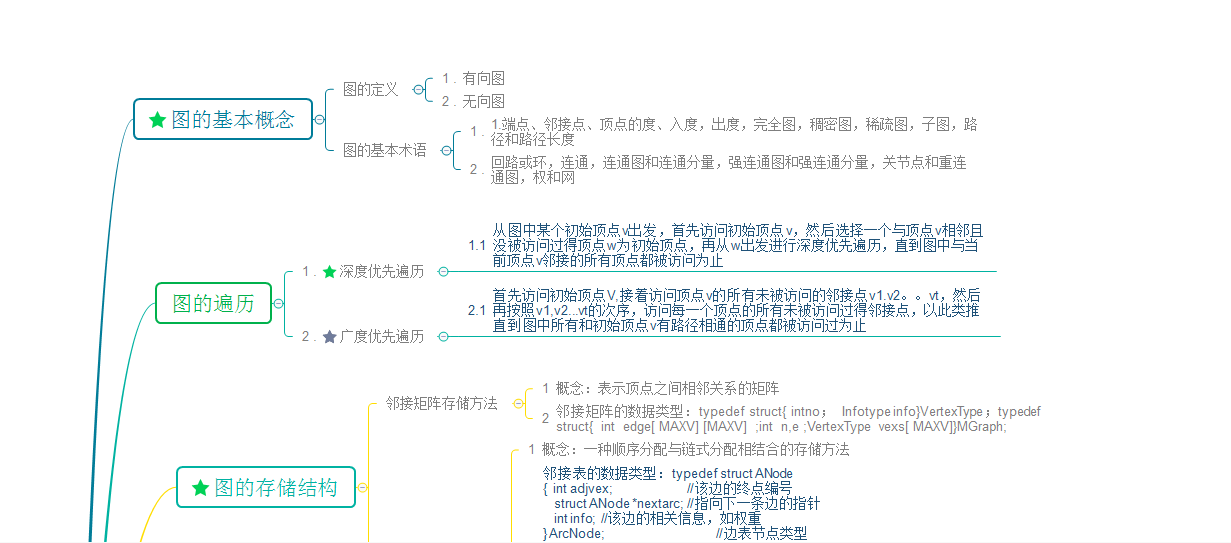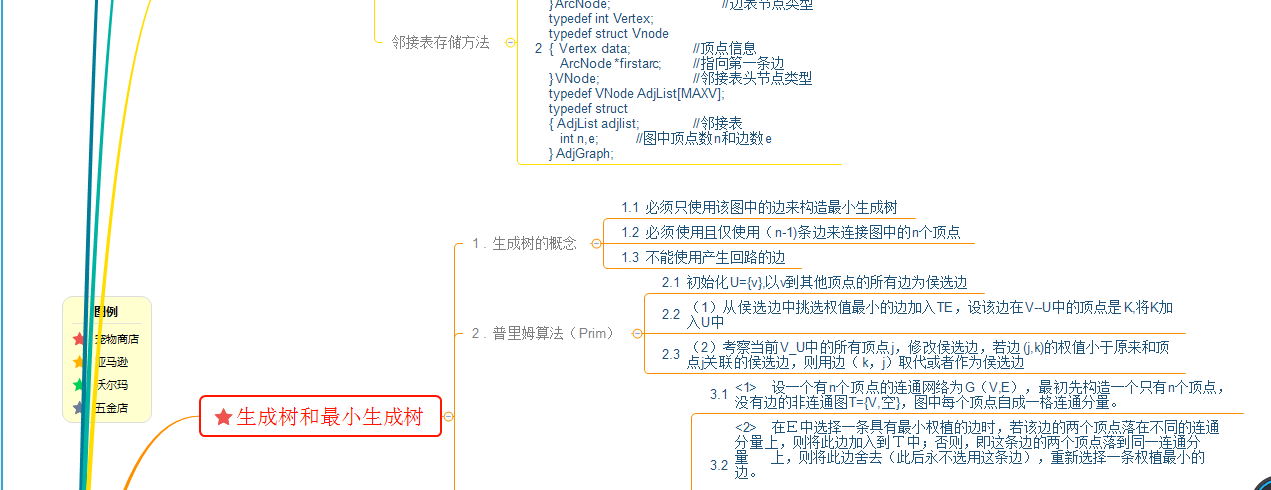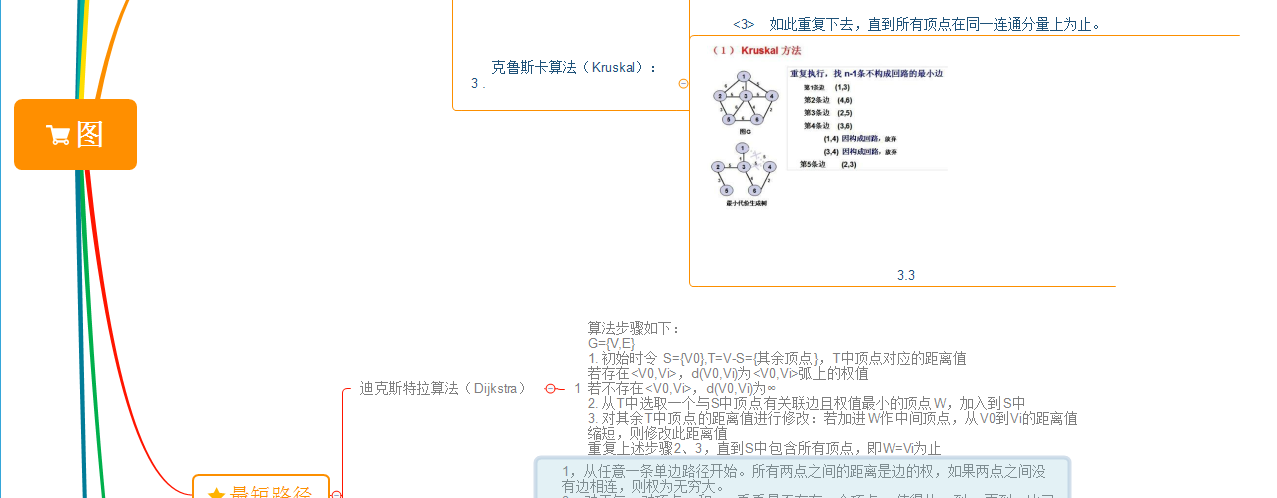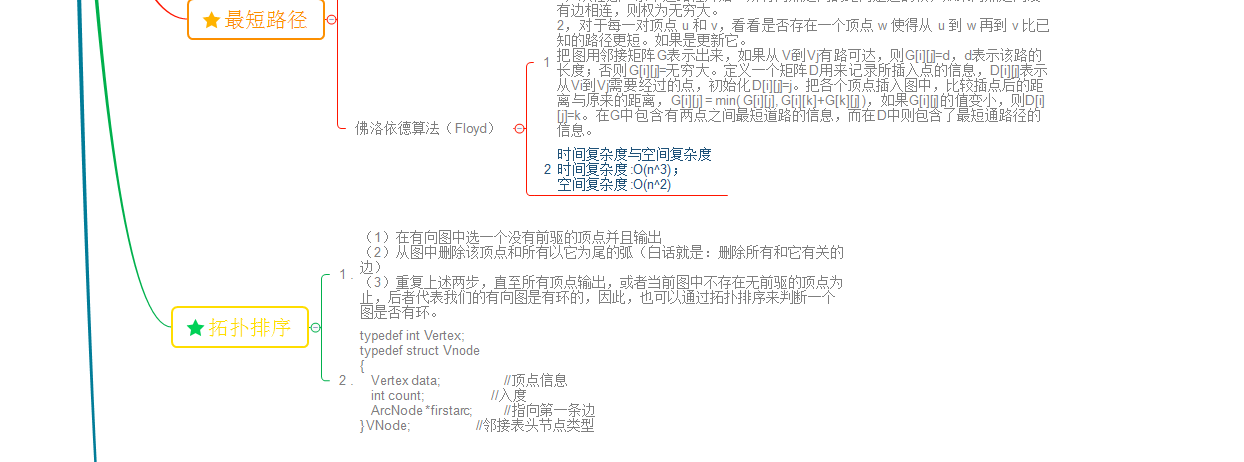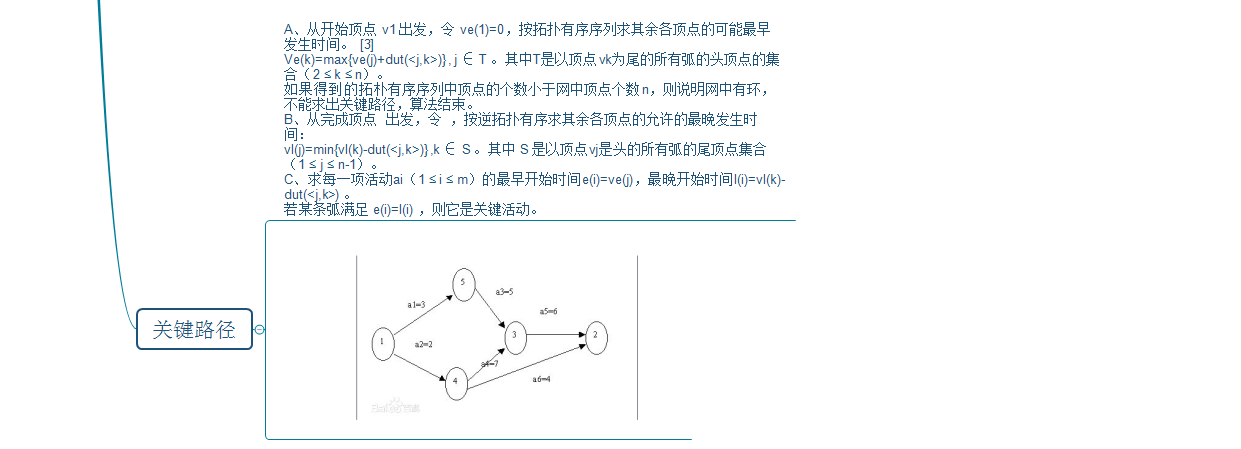## 1.2 图结构学习体会

### 深度遍历的思想：

              ①访问顶点v；


②依次从v的未被访问的邻接点出发，对图进行深度优先遍历；直至图中和v有路径相通的顶点都被访问；
③若此时图中尚有顶点未被访问，则从一个未被访问的顶点出发，重新进行深度优先遍历，直到图中所有顶点均被访问过为止。

### 广度遍历的思想：

               ① 访问顶点vi ；

                  ② 访问vi 的所有未被访问的邻接点w1 ,w2 , …wk ；
③ 依次从这些邻接点（在步骤②中访问的顶点）出发，访问它们的所有未被访问的邻接点; 依此类推，直到图中所有访问过的顶点的邻接点都被访问；


### Dijkstra算法

#### 拓扑排序算法

##### 执行步骤,由AOV网构造拓扑序列的拓扑排序算法主要是循环执行以下两步，直到不存在入度为0的顶点为止。
(1) 选择一个入度为0的顶点并输出之；
(2) 从网中删除此顶点及所有出边。



# 2.PTA实验作业（4分）

## 2.2 设计思路（伪代码或流程图）

定义数组G存放两条路的预算成本
初始化数组G；
for i=1  to N
输入两条道路编号及预算成本
给G赋初值
定义数组lowcost，closest分别用来标记最近顶点已访问过和最少金额
for i=0  to N
在（V-U）中找出离U最近的顶点k,k记录最近顶点的编号
遍历数组G
如果顶点未被访问过且顶点K到顶点的最少金额小于该顶点的预算成本，则将
lowcost[j]=G[k][j];closest[j]=k;

遍历结束将数组中的最少金额相加
end


## 2.3 代码截图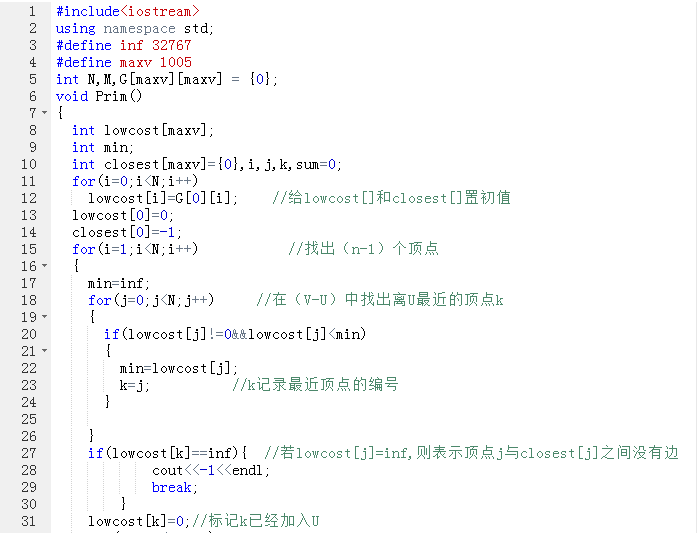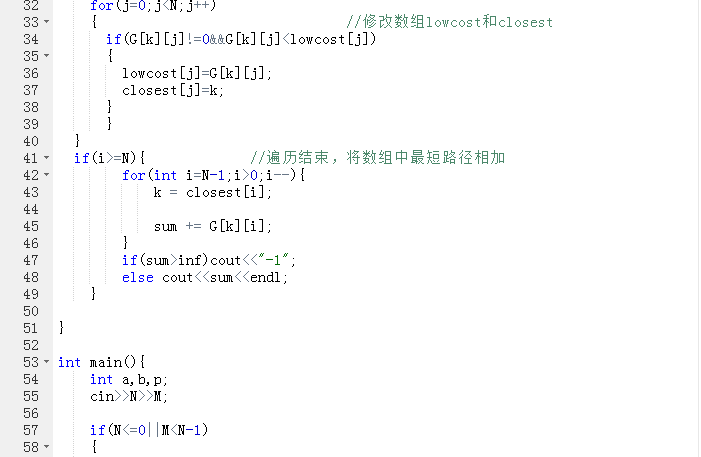## 2.4 PTA提交列表说明。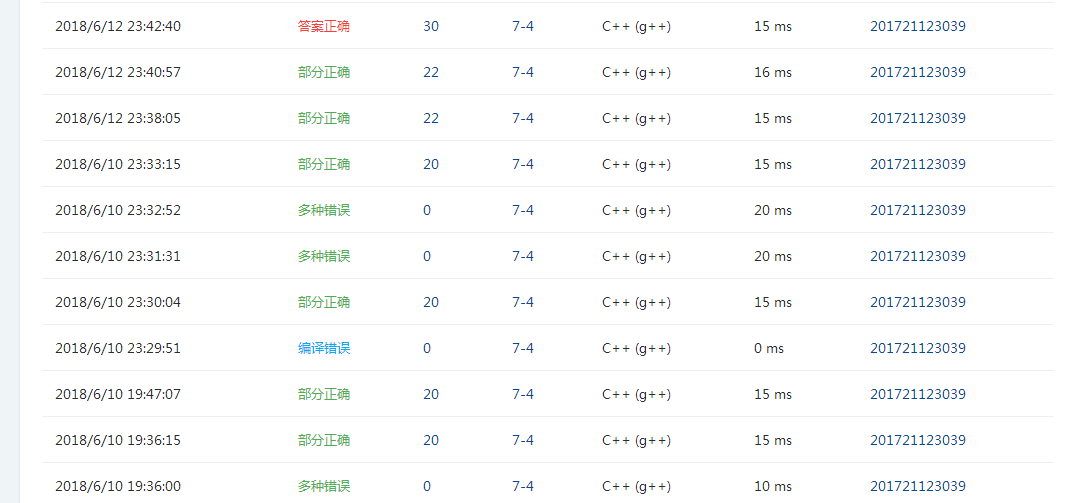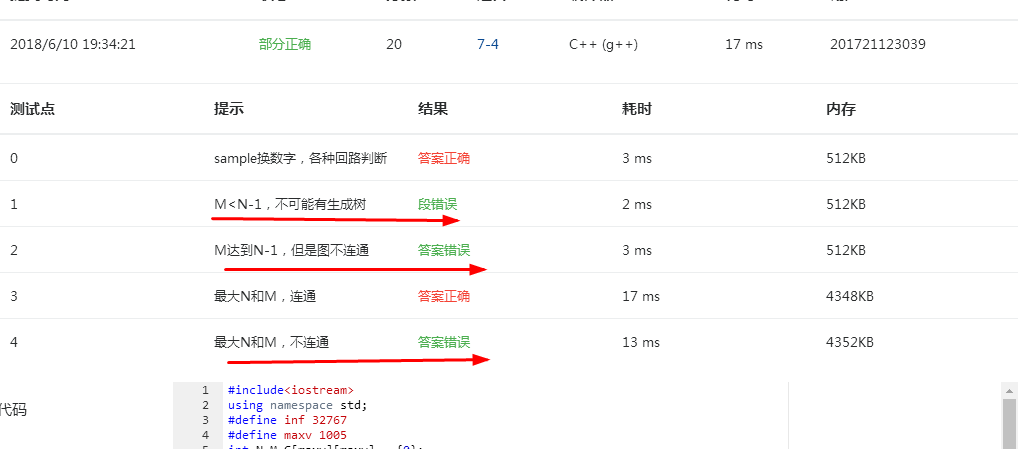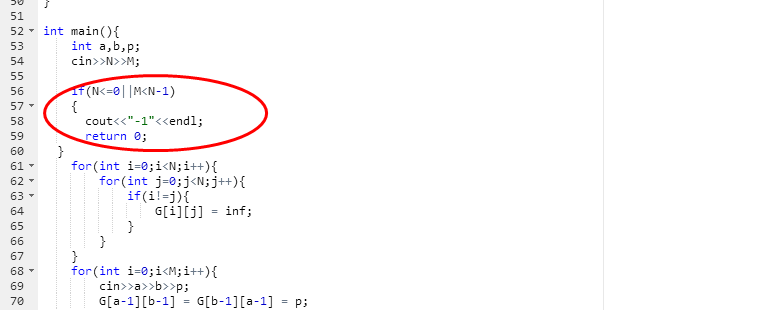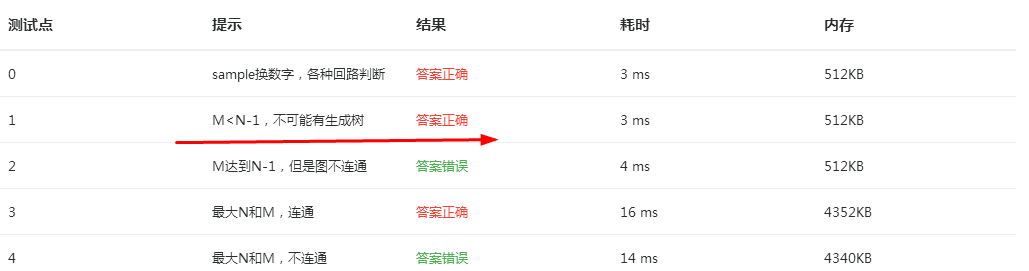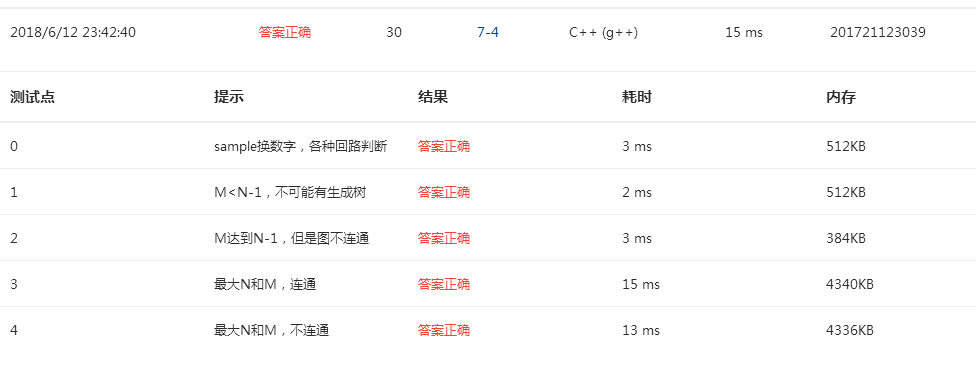## 2.2 设计思路（伪代码或流程图）

定义数组ways;用于储存路径的长度，费用
dist,cost;分别储存由st出发点到个点的最短路径及其费用
visited = {0};储存个点是否加入集合中

for i=0  to M  循环找到最短路径或最少金额
for  j=0  to  M
找到与初始顶点最近的顶点，用mindis保存该顶点
visited标记该顶点已被访问过
for  j=0   to  M
如果顶点未被访问过，且到起始顶点的路径大于最短路径
则将最短路径附给dist[j];同时金额也相应改变
如果距离相等则只改变金额
end


## 2.3 代码截图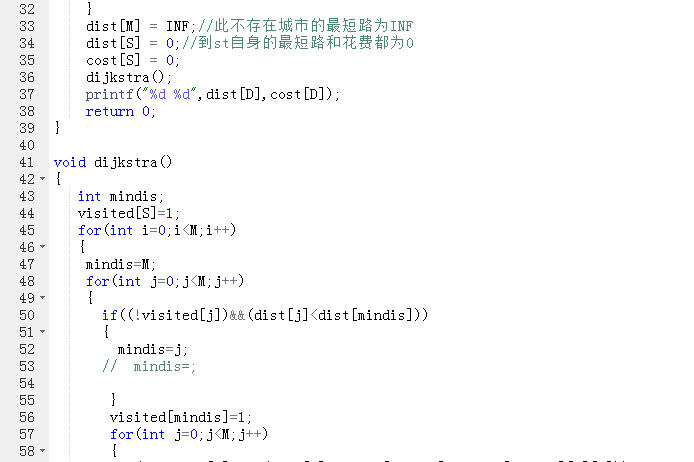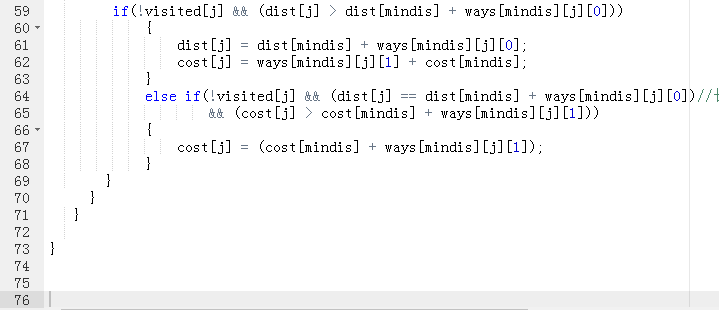## 2.4 PTA提交列表说明。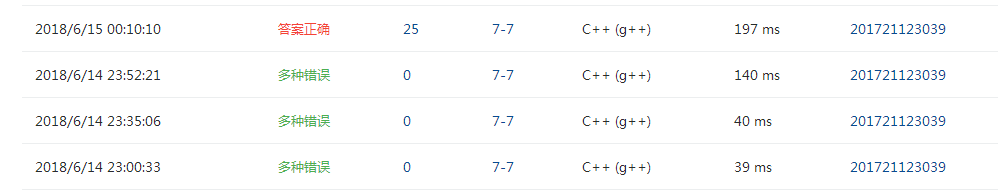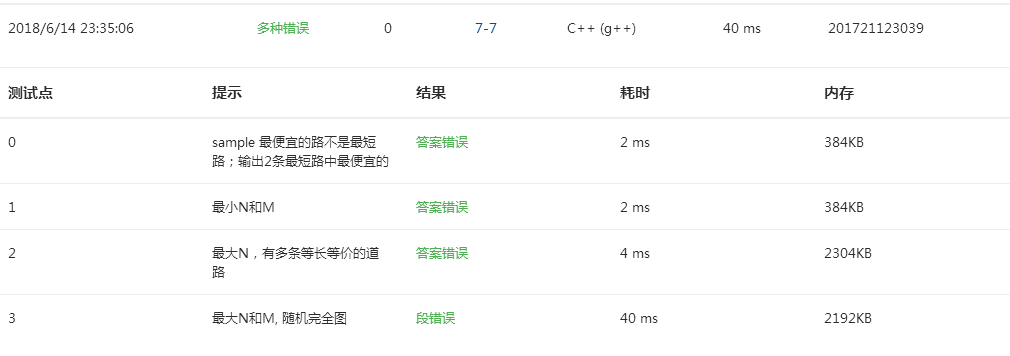## 2.2 设计思路（伪代码或流程图）

定义数组aor存放两个人的关系
while M--
输入两位宾客以及两人的关系数
用数组aor记录两位宾客的关系
if  宾客关系数为1
则将这两位宾客的编号放入并查集
while K--
输入要查阅的宾客编号m，n
如果aor[m][n]=1,则输出No problem
如果aor[m][n]=-1,
如果在并查集中找到m，n，则输出OK but...
否则输出No way
否则
如果在并查集中找到m，n，则输出No problem
否则输出OK
end


## 2.3 代码截图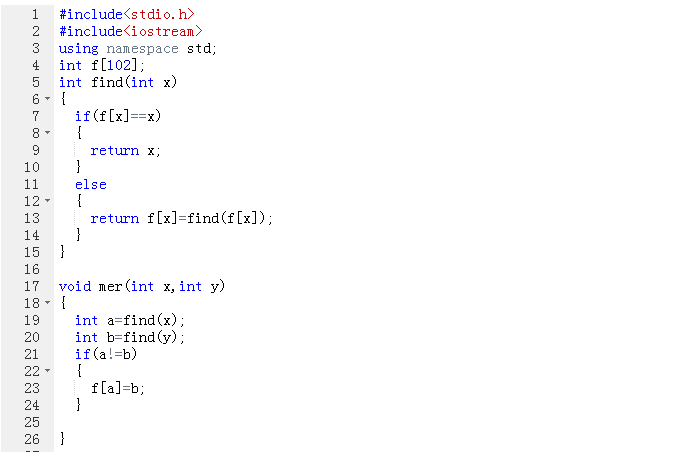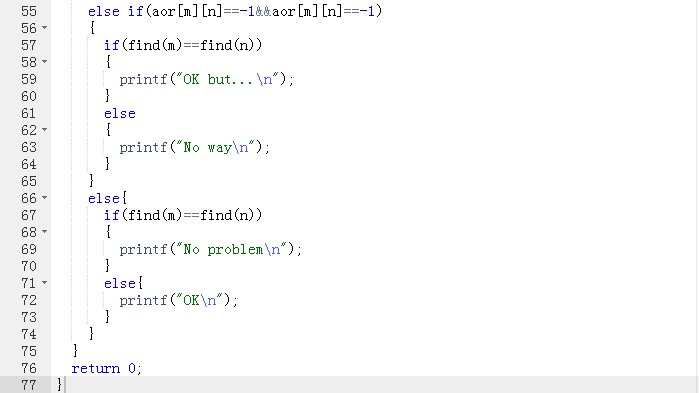## 2.4 PTA提交列表说明。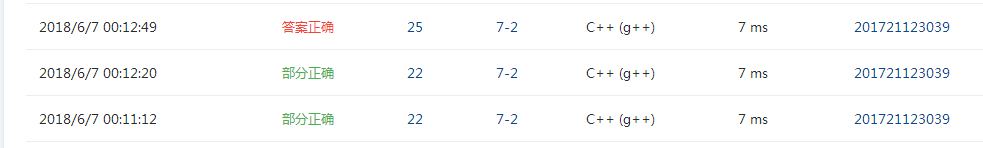# 3.截图本周题目集的PTA最后排名（3分）

## 3.1 PTA排名（截图带自己名字的排名）# 4. 阅读代码（必做，1分）

### 代码：7-8 城市间紧急救援（25 分）

#include <bits/stdc++.h>
#define inf 0x3f3f3f3f
using namespace std;
int N,M,S,D;
int graph,dis,p,sum,ans,theway;
//  邻接矩阵 。最短距离 ，每个点的消防队数目， 最多消防队数目， 最短路径条数，经过此点的最优路径的上一个点
bool vis;  //记录是否访问此点
void dijkstra()
{
ans[S] = 1;
vis[S] = true;
sum[S] = p[S];
int min_poi;

while(1)
{
min_poi = N;
for(int i = 0 ; i < N; ++i)
{
if( !vis[i] && dis[i] < dis[min_poi])
{
min_poi = i;
}
}
if(min_poi == N) break;
vis[min_poi] = true;
for(int i = 0; i < N; ++i)
{
if(!vis[i])
{
if(dis[i] > dis[min_poi] + graph[min_poi][i])
{
dis[i] = dis[min_poi] + graph[min_poi][i];
ans[i] = ans[min_poi];
sum[i] = sum[min_poi] + p[i];
theway[i] = min_poi;
}
else if(dis[i]==dis[min_poi] + graph[min_poi][i])
{
ans[i] += ans[min_poi];
if( sum[i] < sum[min_poi] + p[i])
{
sum[i] = sum[min_poi] +p[i];
theway[i] = min_poi;
}
}
}
}
}
}
int main()
{
int a,b,c;
scanf("%d%d%d%d",&N,&M,&S,&D);
for(int i = 0; i < N; ++i)
for(int j = 0; j < N; ++j)
graph[i][j] = inf;
for(int i = 0; i < N; ++i )
{
vis[i] = false;
scanf("%d",&p[i]);
}
for(int i = 0; i < M; ++i)
{
scanf("%d%d%d",&a,&b,&c);
graph[a][b] = graph[b][a] = c;
}
for(int i = 0; i < N; ++i)
{
dis[i] = graph[S][i];
if(dis[i] != inf) theway[i] = S;
if(S != i && graph[S][i] != inf)
{
sum[i] = p[i] + p[S];
ans[i] = 1;
}
}
dis[N] = inf;
dijkstra();
printf("%d %d\n", ans[D], sum[D]);
int answay,rear = 0;
int thepoi = D;
while(1)                         //   把所求路径上的点储存到数组中，再倒序输出
{
answay[rear++] = theway[thepoi];
thepoi = theway[thepoi];
if(thepoi == S) break;
}
for(int i = (rear - 1); i >= 0; --i)
printf("%d ",answay[i]);
printf("%d",D);
return 0;
}

posted @ 2018-06-17 19:10  千辰烊烊  阅读(154)  评论(0编辑  收藏  举报